# Chapter 11 Motion Section 1 Distance and Displacement

• Slides: 9
Download presentation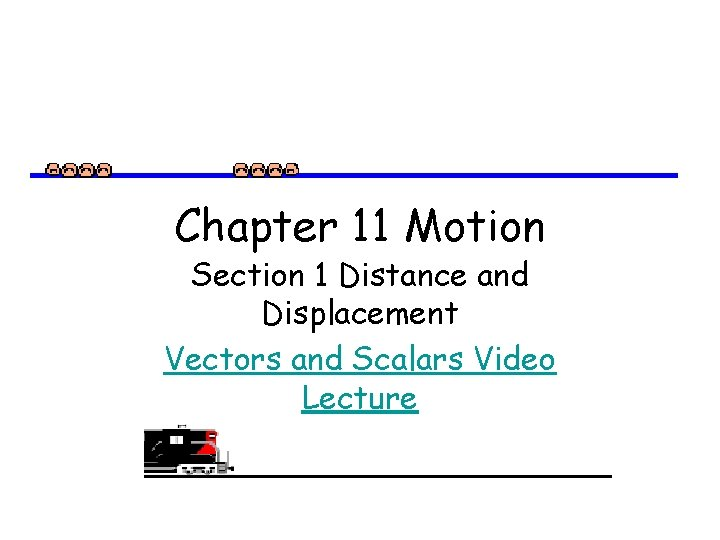Chapter 11 Motion Section 1 Distance and Displacement Vectors and Scalars Video Lecture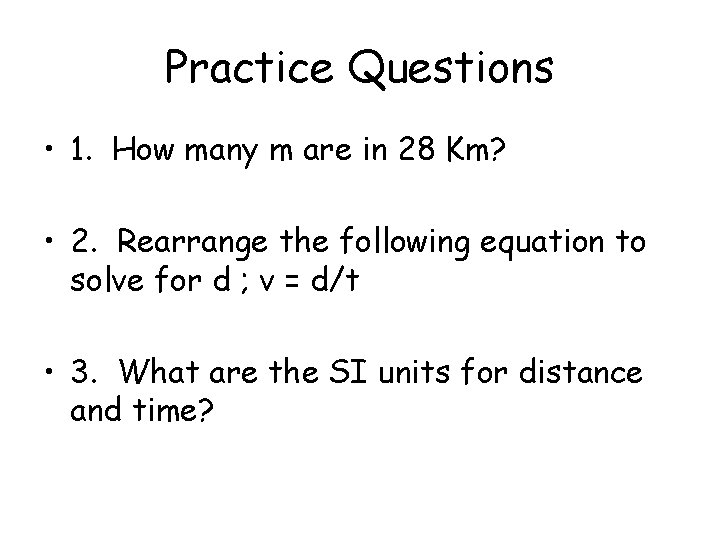Practice Questions • 1. How many m are in 28 Km? • 2. Rearrange the following equation to solve for d ; v = d/t • 3. What are the SI units for distance and time?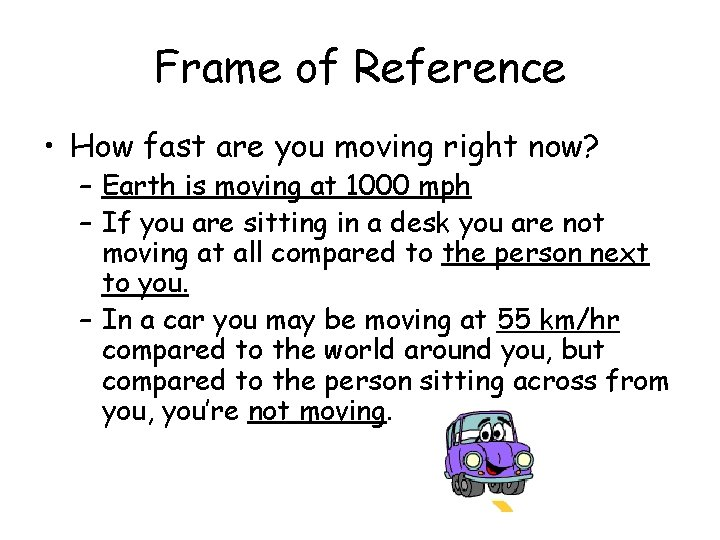Frame of Reference • How fast are you moving right now? – Earth is moving at 1000 mph – If you are sitting in a desk you are not moving at all compared to the person next to you. – In a car you may be moving at 55 km/hr compared to the world around you, but compared to the person sitting across from you, you’re not moving.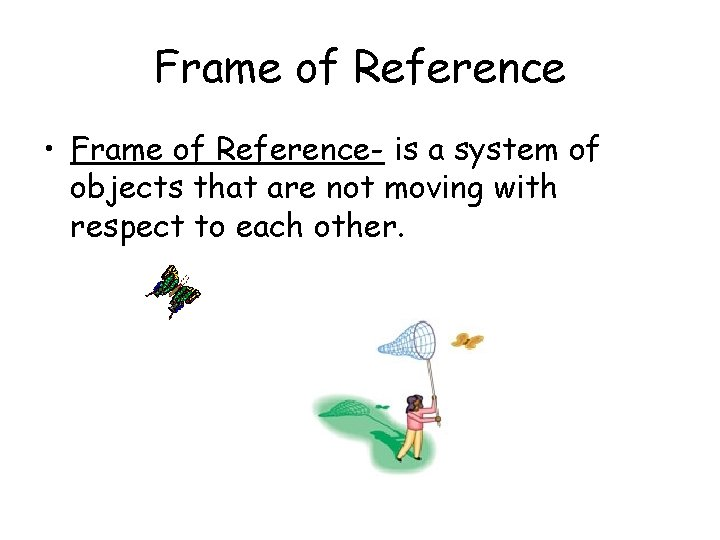Frame of Reference • Frame of Reference- is a system of objects that are not moving with respect to each other.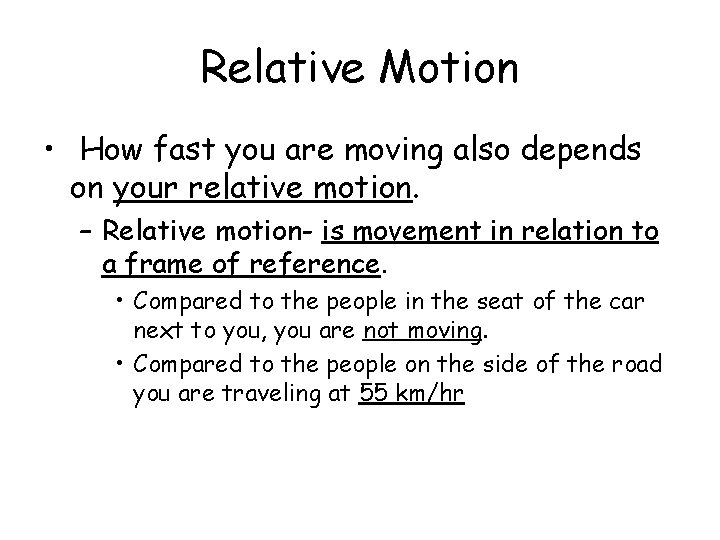Relative Motion • How fast you are moving also depends on your relative motion. – Relative motion- is movement in relation to a frame of reference. • Compared to the people in the seat of the car next to you, you are not moving. • Compared to the people on the side of the road you are traveling at 55 km/hrDistance • Distance- length of a path between two points. – Remember to use appropriate units when measuring distance.Displacement • Displacement is the length of a straight line from the starting point to the ending point and the direction from starting point to ending point. – Example- distance- I walked 5 blocks – Example- displacement- I walked 5 blocks north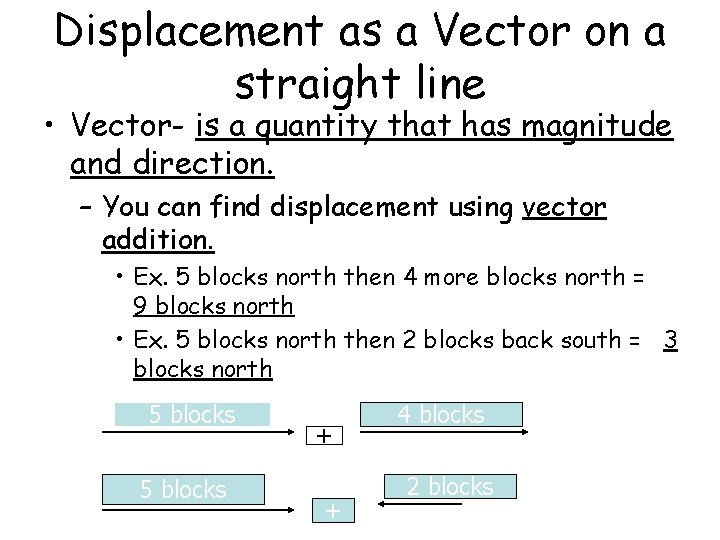Displacement as a Vector on a straight line • Vector- is a quantity that has magnitude and direction. – You can find displacement using vector addition. • Ex. 5 blocks north then 4 more blocks north = 9 blocks north • Ex. 5 blocks north then 2 blocks back south = 3 blocks north 5 blocks + + 4 blocks 2 blocksDisplacement not along a straight line • If you graph the total distance and draw a straight line from the starting point to the finishing point you can find the total displacement